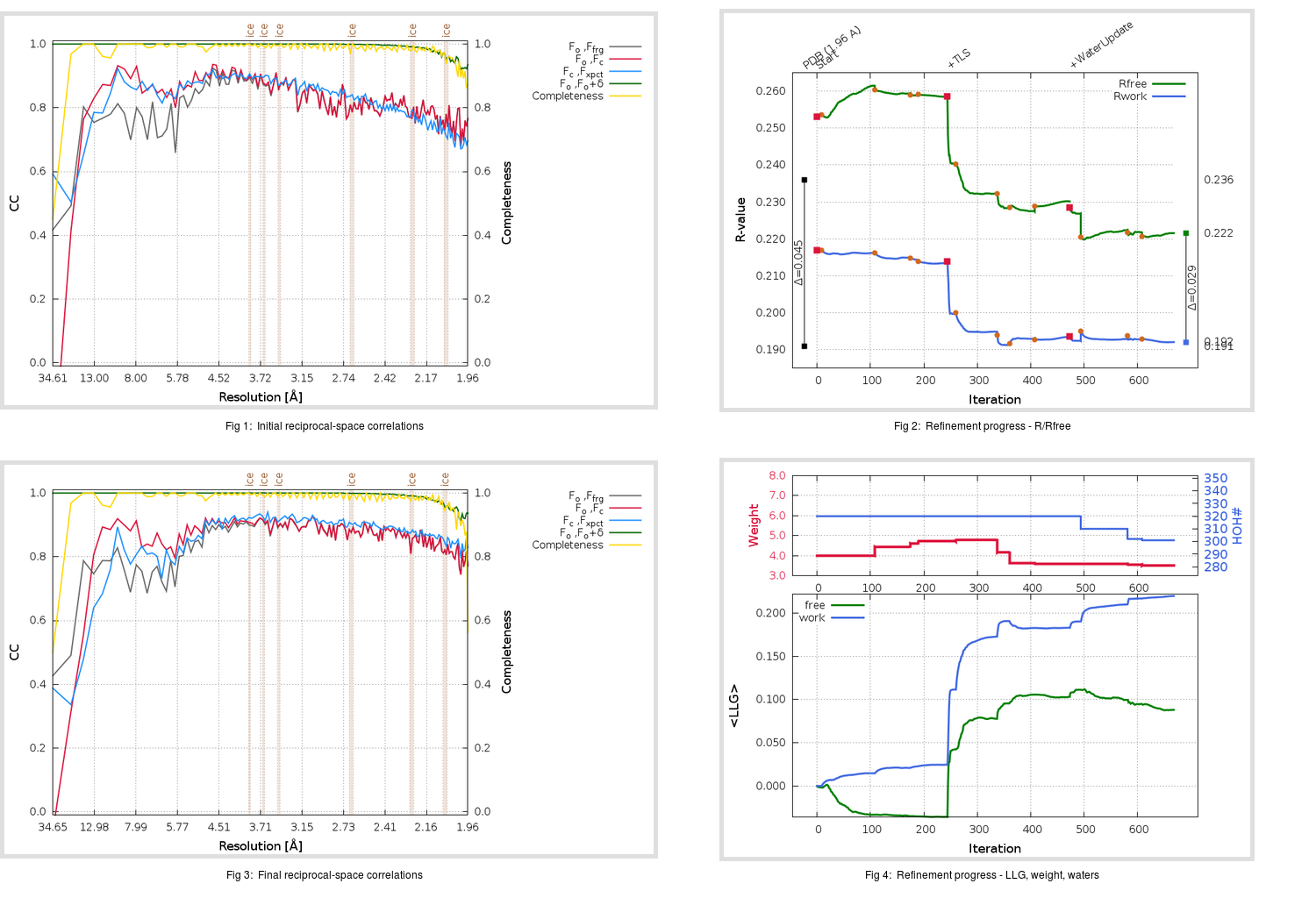Content:

## Deposited

` `
 Date deposited Date data collection Resolution R, Rfree 20111213 20100310 1.96 0.1890 0.2360

Molprobity (CCP4 7.0 version) summary:

```Ramachandran outliers =   0.66 %
favored =  97.36 %
Rotamer outliers      =   4.40 %
C-beta deviations     =     1
Clashscore            =   5.12
RMS(bonds)            =   0.0104
RMS(angles)           =   1.27
MolProbity score      =   1.88
Resolution            =   1.96
R-work                =   0.1890
R-free                =   0.2360
```

```Number of waters      =   320

<B> (all atoms) =   45.91 ( sd =   14.96 ) for       2772 non-hydrogen atoms
<B>   (protein) =   44.87 ( sd =   15.15 ) for       2412 non-hydrogen atoms
<B>     (water) =   52.99 ( sd =   11.13 ) for        320 non-hydrogen atoms
<B>    (others) =   50.66 ( sd =   12.70 ) for         40 non-hydrogen atoms

B min/max       (all non-hydrogen atoms) =   22.50 /  154.06
B min/max   (protein non-hydrogen atoms) =   24.81 /  154.06
B min/max     (water non-hydrogen atoms) =   22.50 /   84.76
B min/max     (other non-hydrogen atoms) =   31.84 /   83.89
```

## BUSTER (re-)refinement

` `

Molprobity (CCP4 7.0 version) summary:

```Ramachandran outliers =   0.66 %
favored =  97.69 %
Rotamer outliers      =   4.40 %
C-beta deviations     =     0
Clashscore            =   3.07
RMS(bonds)            =   0.0116
RMS(angles)           =   1.57
MolProbity score      =   1.65
Resolution            =   1.96
R-work                =   0.1921
R-free                =   0.2216
```

```Number of waters      =   301

<B> (all atoms) =   46.88 ( sd =   12.99 ) for       2753 non-hydrogen atoms
<B>   (protein) =   45.09 ( sd =   11.69 ) for       2412 non-hydrogen atoms
<B>     (water) =   60.06 ( sd =   14.03 ) for        301 non-hydrogen atoms
<B>    (others) =   53.93 ( sd =   15.85 ) for         40 non-hydrogen atoms

B min/max       (all non-hydrogen atoms) =   24.09 /  104.48
B min/max   (protein non-hydrogen atoms) =   25.88 /   91.52
B min/max     (water non-hydrogen atoms) =   24.09 /  104.48
B min/max     (other non-hydrogen atoms) =   39.02 /   95.14
```

Refinement progression:Results:

` `
 File Remark 3V3M_aB_refine.01_03_refine.pdb.gz exact refinement commands are in header 3V3M_aB_refine.01_03_refine.mtz.gz including original deposited data and several re-refinement map coefficients 3V3M_aB_refine.01_03_BUSTER_model.cif.gz including any non-standard compound restraints 3V3M_aB_refine.01_03_BUSTER_refln.cif.gz